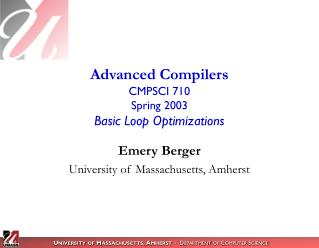DownloadDownload PresentationAdvanced Compilers CMPSCI 710 Spring 2003 Basic Loop Optimizations

# Advanced Compilers CMPSCI 710 Spring 2003 Basic Loop Optimizations

Download Presentation## Advanced Compilers CMPSCI 710 Spring 2003 Basic Loop Optimizations

- - - - - - - - - - - - - - - - - - - - - - - - - - - E N D - - - - - - - - - - - - - - - - - - - - - - - - - - -
##### Presentation Transcript

1. Advanced CompilersCMPSCI 710Spring 2003Basic Loop Optimizations Emery Berger University of Massachusetts, Amherst

2. Topics • Last time • Optimizations using SSA form • Constant propagation & dead code elimination • Loop invariant code motion • This time • Loop optimizations • Induction variable • Linear test replacement • Loop unrolling • Scalar replacement

3. Easy Detectionof Loop Induction Variables • Pattern match & check: • Search for “i = i + b” in loop • i is induction variable if no other assignment to i in loop • Pros & Cons: • Easy! • Does not catch all loop induction variablese.g., “i = a * c + 2”

4. Taxonomy of Induction Variables • basic induction variable: • only definition in loop is assignmentj = j § c, where c is loop invariant • mutual induction variable: • definition is linear function of other induction variable i‘: • i = c1 * i‘§ c2 • i = i‘ / c1 § c2 • family of basic induction variable j: • set of induction variables i such that i is always assigned linear function of j

5. Strength Reduction • Replace “expensive” op by “cheaper” one • E.g., replace multiply by addition • Apply to induction variable families • Especially: array indexing

6. Strength Reduction Algorithm • Let i be induction variable in the family of basic induction variable j: • i = c1 * j + c2 • Create new variable i’ • Initialize in pre-header: i’ = c1*j + c2 • Track value of j: after j = j + c3, add i’ = i’ + (c1 * c3)

7. Strength Reduction Example

8. Candidates for Strength Reduction • Induction variable IV multiplied by invariant • Recursively: • IV * IV, IV mod constant, IV + IV

9. Strength Reduction Algorithm

10. Strength Reduction Examples • basic induction variable: • only definition in loop is assignmentj = j § c, where c is loop invariant • mutual induction variable: • definition is linear function of other induction variable i‘: • i = c1 * i‘§ c2 • i = i‘ / c1 § c2 • family of basic induction variable j: • set of induction variables i such that i is always assigned linear function of j

11. Linear Test Replacement • Eliminates induction variable! • After strength reduction, loop test is often last use of induction variable • Algorithm: • If only use of IV is loop test and its own increment, and test is always computed • i.e., only one exit from loop • Replace test with equivalent one: • E.g., “i comp k” ) “i_50 comp k*50”

12. Linear Test Replacement Example

13. Loop Unrolling • To reduce loop overhead, we can unroll loops • Advantages: • Execute fewer total instructions • More fodder for common subexpression elimination, strength reduction, etc. • Move consecutive access closer together • Disadvantages: • Code bloat • Still updating through memory

14. Scalar Replacement • Problem: register allocators never keep a[i] in register • Idea: trick allocator • Locate patterns of consistent reuse • Replace load with copy into temporary • Replace store with copy from temporary • May need copies at end of loop • E.g., when reuse spans > 1 iteration • Advantages: • Decreases number of loads and stores • Keeps reused values in registers • Big performance impact (2x, 3x!)

15. Scalar Replacement Example • Scalar replacement exposes the reuse of a[i] • Traditional scalar analysis – inadequate • Use dependence analysis to understand array references (later)

16. Next Time • Common Subexpression Elimination • Read ACDI: • Ch. 12, pp. 343-355 • Ch. 13, pp. 378-396